telescopeѲptics.net          ▪▪▪▪                                             CONTENTS

4.2. ComA OPTICAL ABERRATION

In general, coma wavefront aberration occurs either due to the incident wavefront being either tilted, or decentered with respect to the optical surface. Hence, it is either an aberration affecting off-axis image points, or the  result of lateral misalignment of optical surfaces, respectively. Important difference between the two is that with the former coma takes form of an off-axis aberration increasing with the field angle, and with the latter it is of even magnitude across the field, including field center.

The form of coma most often dominating in amateur telescopes is the lower (4th) order or primary coma. As illustrated on FIG. 39, its wavefront deviation has reverse symmetry along the axis of aberration, with one side flatter, and the other more curved with respect to its perfect reference sphere.FIGURE 39: TOP - Primary coma wavefront aberration. RIGHT: incident wavefront Wi, tilted in regard to the optical surface, loses the symmetry needed to become spherical after reflection; as a result, rays originating at the actual wavefront scatter, forming coma blur. Reflected wavefront Wa deviates from the reference sphere WG centered at the Gaussian image point G; after correcting for tilt, better reference sphere WP is found centered at the best (diffraction) focus Fb. LEFT, an exaggerated illustration of the coma wavefront deformation, and how it causes rays to spread into coma  blur (for clarity, the blur is rotated 90°). The cross-section of the middle (solid blue) and the edge side projection (dashed blue) of the comatic wavefront indicate the form of its deviation from the perfect reference sphere (red dots). It has an element of tilt combined with lobe-like deformations on the opposite sides of wavefront. Only the central spot of the wavefront is nearly spherical, focusing at the point of highest intensity Fb (best, or diffraction focus location), which is located at 2/3 of the sagittal coma, along the axis of aberration. The RMS spot radius is the smallest when calculated at the PSF centroid C, when it is 0.29 of the tangential coma. It is obvious that as the wavefront error - and the blur size relative to the Airy disc - increase, best focus location shifts farther away from the Gaussian image point.
BOTTOM - Cross-sections, wavefront map and 3-D wavefront illustration of primary coma (from Wyant).Mapping rays scattered by coma wavefront deformation follows simple, elegant symmetry. As illustrated on FIG. 40, every zone in the pupil focuses not into a point, but into a circle of the diameter

R = hz2/2f2             (11)

with h being the linear height in the image plane, z=ρd=ρD/2 the zonal height in the pupil (D is the pupil, or aperture diameter and ρ the zonal height normalized to 1), and f the focal length. The circle is formed by each pair of diametrically opposed points on a given pupil zone focusing into a single circle point. The center of each circle is also shifted along the axis of aberration, away from the Gaussian image point by a length equal to the circle diameter.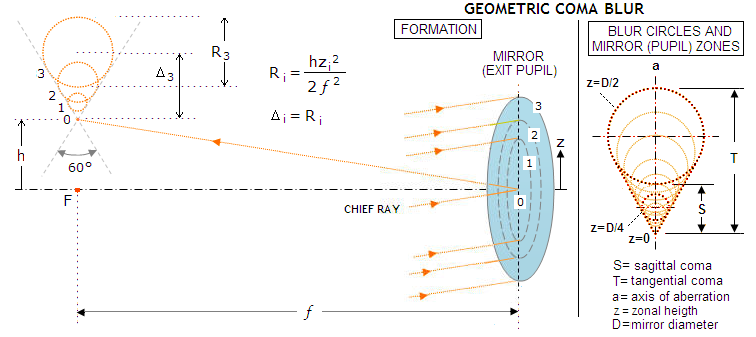FIGURE 40
: Geometry of the coma ray spot diagram for primary coma, created by rays projected from the coma wavefront reflected from mirror surface. The spot consists entirely from progressively expanding circles, with the exception of the very central ray, projecting into the tip of the sagitta. Both, the diameter R of the coma blur circles formed in the focal zone and their shift Δ from the Gaussian focus increase with the square of the pupil (zonal) height z, expanding around the axis of aberration a, in the direction of the axis. Diameter of the largest coma blur circle, formed by the very edge of the aperture, is 2/3 of the blur length. The ray circle formed by the 0.5 (z=D/4) wavefront zone is only 1/4 of the diameter of of the edge circle (z=D/2), and has 1/4 of its shift. Tangential coma (T) is the entire blur length, with the sagittal coma (S=T/3) being the "V" shaped tip oriented toward Gaussian image point. About 80% of all rays are contained within the sagittal part of the coma blur. It is the sagittal part of the comatic point image that is actually visible as a deformation of the point-source image in a telescope.

The wavefront aberration function for primary coma at the Gaussian image point is given in Table 4. But best focus location is shifted laterally from this point, i.e. requires correction for the wavefront tilt. Aberration function (i.e. the wavefront error as optical path difference with respect to a reference sphere) for coma at the best focus is given by: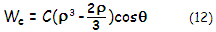with C being the coma peak aberration coefficient, ρ the pupil radius normalized to 1 and θ the pupil angle. Note that Wc at its maximum represents 1/2 of the P-V wavefront error, since it gives identical value of opposite sign for negative ρ (i.e. for the opposite half of pupil radius). As shown at left, the aberration peaks for ρ=1 and θ=0° and 180° - i.e. along the axis of aberration - with the sign of aberration changing symmetrically on both sides of the wavefront (except for θ=0, when deviation is zero across the pupil radius). Second factor in the brackets - effectively correction for wavefront tilt - makes the P-V coma error at the best focus ("balanced" coma, shown at left) three times smaller at its maximum than the P-V error at the paraxial (Gaussian) focus. The latter is referred to as "classical", or Seidel coma, where the P-V wavefront error is given by W=Cρ3cosθ (FIG. 42, middle).

The RMS wavefront error for coma at the best focus, in terms of the peak aberration coefficient C is:

ωc = C/720.5          (16)

In terms of the P-V wavefront error it is ωc=2Wc/32, with Wc being, as given above, the coma peak wavefront error (half of the P-V error).

On FIG. 39, the peak error on the top radius (edge point) is positive, being farther to the center of a perfect reference sphere than its perfect reference point, while the peak error on the bottom radius is negative. This is so called "positive" coma, with the top section of the wavefront flattened with respect to the reference sphere, and the bottom portion more curved. Its comatic tail is oriented outward, away from field center. Presence of the pupil angle factor (cosθ) indicates that the aberration is not rotationally symmetrical. The peak aberration coefficient is given by:

C = cαd3           (13)

with c being the coma aberration coefficient (simplified notation for 1w31 from Table 4), α the field angle (in radians) and d the pupil radius.

For general optical surface, coma aberration coefficient is given by:

c=0.5n2[(1/O)-(1/R)][(1/n'I)-(1/nO)]

where I, O are the image and object distance, and n, n' are the refractive index of incidence and refraction or reflection, respectively.

Coma aberration coefficient c for either refractive or reflective surface, for object at infinity, and aperture stop at the surface is given by: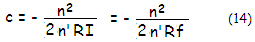with f being the focal length, and n and n' being the index of incidence and refraction/reflection, respectively.

For a single thin lens with the stop at the surface, coma coefficient is: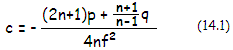with p, q and f being the lens position factor, shape factor and focal length, respectively. Thin lens is coma-free if its shape factor q and position factor p relate as q=-(2n+1)(n-1)p/(n+1). Lens doublet can have both, coma and spherical aberration cancelled. For given shape p and q, the sign of coma is not affected by lens power, i.e. it is the same for positive and negative lens. However, if placed one after another, their respective p values - and possibly sign as well - will be different, and so will the coma induced by each element.

With regard to the aperture stop position, it does affect coma only with objectives not corrected for spherical aberration. With an aplanatic (corrected for spherical aberration and coma for infinity) lens system, stop position doesn't affect neither coma, nor astigmatism and field curvature.

For mirror surface, the coma coefficient for any object distance is given by: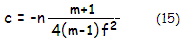with m being the transverse image magnification (defined following Eq. 9.). For distant objects m=0, n=1 for mirror in air oriented to the left, and the coefficient reduces to: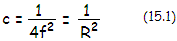As the aberration function shows, deviation from the perfect reference wavefront is largest for ρ=1 and either cosθ = 1 or cosθ = -1 (i.e. θ=0 or θ=π radians, respectively). With θ being the position angle in the pupil, measured to the plane of the axis of aberration, the maximum deviation occurs at the marginal points of the wavefront in the plane of aberration (FIG. 25, left). The deviation is zero for cosθ=0 (i.e. θ=π/2 or θ=3π/2), which is the wavefront diameter orthogonal to the plane of aberration. For ρ smaller than |~0.8|, the deviation changes the sign, as a result of the two lobe-like deformations, illustrated on FIG. 39, left.

As mentioned, the above relations for coma aberration are for a mirror with the aperture stop at the surface. For displaced stop, the coma aberration coefficient c changes with a factor Δ[ c'=1-(1+K)σ]/σR;, with Δ being the chief ray height on the mirror (given by a product of the mirror-to-stop separation and incident angle), K being the mirror conic and σ the mirror-to-stop separation in units of the mirror radius of curvature, numerically positive. Thus, for displaced stop, the aberration coefficient for object at infinity is: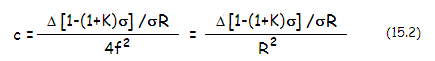Since Δ and σ change in proportion to the stop separation, stop position doesn't influence coma of a paraboloid (K=-1), while cancels coma of any conic K>-1 for the stop separation σ=1/(1+K). On the other hand, displaced stop increases coma for K<-1 (hyperboloid).

This applies for objects at infinity; for close objects for which the mirror object-image magnification m significantly differs from zero, the change factor in the coma coefficient caused by the aperture stop displacement is obtained by replacing both unit figures in the "infinity" object distance factor c' with (m+1)/(m-1), or (1-Ω), Ω being the reciprocal of the object distance in units of the radius of curvature, numerically positive. It gives the coefficient as: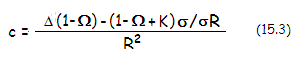Note that (1-Ω) is just a different form of (1-2ψ) parameter, used in Eq. 9.1, ψ being the reciprocal of the object distance O in units of the focal length, ψ=f/O.

The wavefront error changes with the coefficient. Considering that the magnification m is negative, coma diminishes with object distance, dropping to zero for m=-1 (for object at the mirror center of curvature) and stop at the surface (σ=0), regardless of the conic.

Due to the peak wavefront deviations with coma affecting relatively small area, the error averaged over the entire wavefront is smaller for coma than for spherical aberration for any given P-V wavefront error (FIG. 41).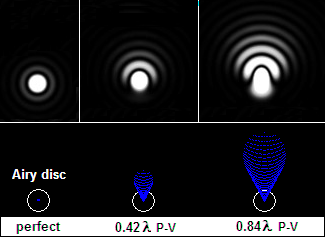FIGURE 41: Coma ray aberration as a ray spot (bottom) and the actual diffraction pattern (top).  Top: from left to right, perfect diffraction pattern of a relatively bright star, diffraction pattern affected with 0.42 wave P-V wavefront error of coma (corresponding to 0.074 wave RMS, for 0.80 Strehl, thus comparable to 1/4 wave P-V of primary spherical aberration), and diffraction pattern affected by 0.84 wave P-V wavefront error of coma (~0.15 wave wavefront error RMS, comparable to 1/2 wave P-V of spherical aberration). As the error increases, best focus location shifts from the middle of the Airy disc in the direction of intensity shift. Unlike spherical aberration, there is no gain from axial defocus, which is pretty much obvious from the shape of wavefront deformation. Bottom: corresponding ray spot plots for identical amounts of the aberration. The "V" shaped form obvious in the ray spot doesn't become apparent in the actual diffraction pattern until the error exceeds ~1 wave P-V, provided there is sufficient magnification to make it visible to the eye.

For small levels of the aberration, the shift from the Gaussian image point to the best focus location is transverse, in the image plane and along the axis of aberration, given by 4FC/3=8FWc/9. That places best focus at 1/4.5 of the blur length away from the Gaussian image point (the tip). The shift effectively corrects for the wavefront tilt element. Unlike spherical aberration, the peak aberration coefficient C for coma does not equal the p-v wavefront error. At the location of best focus, the P-V wavefront error is 2C/3, with the peak wavefront error being ±C/3. The error is smaller by a factor of 3 than at the location of Gaussian image point, for which the tilt is not corrected.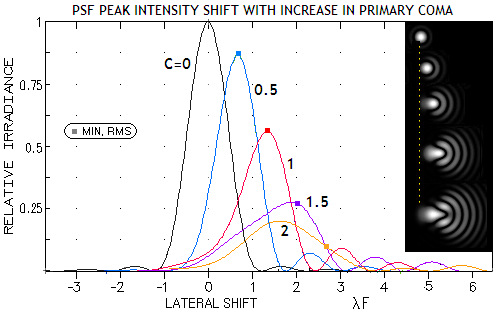It should be mentioned that with coma, as with aberrations in general, the lowest RMS wavefront error produces the highest PSF peak only as long as the magnitude of aberration remains relatively small. In general, under 0.15 wave RMS. Specifically for coma, the PSF peak shifts away from the best focus (with respect to the magnitude of wavefront error), as defined above, as the aberration exceeds 0.76 wave P-V (0.134 wave RMS). For larger errors, the PSF peak is located generally closer to the Gaussian focus, but its exact location varies with the error magnitude (left).

Simulations of the actual PSF at right show the shift in 2-D. It occurs along the axis of aberration (or, in terms of the geometric ray spot, along its axis of symmetry), hence the magnitude of shift diminishes from its maximum for line of sight perpendicular to the axis, to zero ifor line of sight coinciding with the axis of aberration.

Coma transverse aberration also can be expressed in terms of the peak aberration coefficient C. Tangential coma is given by T=6fC/n'D, f being the focal length and D the aperture diameter. For mirror oriented to the left, n'=-1, and T=6FC, with F being the focal ratio number, F=f/D. With the peak aberration coma coefficient C=αD/32F2 for distant objects, tangential coma can be expressed directly as: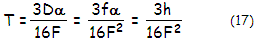as transverse aberration (h=αf, the linear height in the image plane), and

Ta = T/f = 3h/16fF2 = 3h/16DF3 = 3α/16F2          (17.1)

as angular tangential coma (in radians). In terms of peak coma wavefront error Wc, T=18FWc (with the peak wavefront error being, as mentioned, 1/2 of the P-V error).

From Eq. 11, tangential coma changes in proportion to the square of the pupil (zonal) height, or with ρ2 for the pupil radius normalized to 1. As already mentioned, sagittal coma S=T/3; it is larger than the P-V wavefront error by a factor of 3F. Geometric coma blur (tangential coma) can also be expressed in terms of the RMS wavefront error ω, as t=ω2592/2.44, in Airy disc diameters; also, in terms of the peak aberration coefficient as t=6C/2.44 (in Airy disc diameters), for the RMS wavefront error ω and peak aberration coefficient C in units of the wavelength. For any given RMS wavefront error, tangential coma is smaller than spherical aberration blur at the best focus by a factor (81/360)1/2.

Finally, the RMS ray spot radius for balanced coma is given by rRMS=27/9FC, which in terms of the tangential coma becomes 7/9T/3. In other words, it is smaller than the tangential coma blur by a factor 0.294. The coma RMS blur radius is smallest when measured around coma's PSF centroid (at 1/3 of the tangential coma, along the axis of aberration; C on Fig. 39 left). The smallest RMS blur radius is given by r'RMS=8/3FC, or smaller by a factor of 0.926 than the RMS blur radius centered at the diffraction focus. However, due to the increase in wavefront tilt error component, the P-V error of coma for the wavefront centered at the PSF centroid is at the minimum for ρ=1/3, and for the aberration function Wc= C(ρ3-ρ)cosθ. This gives the peak wavefront error Wc=-0.3849C, greater by a factor of 1.15 than the peak wavefront error - and RMS wavefront error - at the best focus.

In units of Airy disc diameter, the RMS blur diameter at the best focus is RRMS=27/9C/1.22, with the peak aberration coefficient C in units of the wavelength. Since the P-V wavefront error of coma W=2Wc=2C/3, it can also be written as RRMSW7/1.22. In terms of coma RMS wavefront error ωc, it is RRMS=414ωc/1.22.

EXAMPLE: For a 200mm f/5 paraboloid, thus d=100 and R=-2000, the peak wavefront error of coma at 1.4mm off-axis (with the field angle α=1.4/1000=0.0014), setting ρ=1 and θ=0 in Eq. 12, comes to C/3. With the peak aberration coefficient C=cαd3=αd3/R2=0.00035mm, the peak wavefront error of coma is Wc=0.0001167mm. The P-V wavefront error is twice as much, or 0.000233mm. In units of 550nm (0.00055mm) wavelength, it is 0.424 (1/2.36 wave). The RMS wavefront error is ω=C/72=0.000041mm or, in units of 550nm wavelength, 0.075 (1/13.33 wave). The RMS wavefront error can also be obtained from the P-V wavefront error as ω=2Wc /32. Either way, the aberration is at the conventional "diffraction-limited" level.

The transverse tangential coma T=6FC=0.0105mm, or 1.56 Airy disc diameters. Therefore, angular tangential coma Ta=T/f is 0.0000105 in radians, or 206.265x0.0000105=2.17 arc seconds. Since both, wavefront error and geometric (ray) aberrations are directly proportional to the aberration coefficient, it implies that they are in a constant proportion themselves. In other words, doubling the wavefront error also doubles the geometric aberration.

The RMS blur radius rRMS=27/9FC=0.000617mm, and the RMS blur diameter in units of the Airy disc diameter RRMS=27/9C/1.22=0.92, for C in units of the wavelength.

Analogous to the higher-order spherical aberration, strongly curved surfaces generate higher-order (secondary) coma, similar in appearance to its lower-order form, but not identical. Unlike primary coma, where the zonal ray circles increase with the square of zonal height, and the circle center is at its diameter away from Gaussian focus, with secondary coma the zonal ray circle diameter increases with the fourth power of zonal height, while its center shifts by 1.5 the circle radius from Gaussian focus. As a consequence, the cone formed by these circles is wider, outlining a 90 angle (FIG. 42). The ray geometry indicates that secondary coma displaces relatively less energy into the outer portion of the blur, with the sagittal coma being only 1/4 of the tangential coma (blur length). Accordingly, the resulting diffraction image would have less noticeable comatic tail, and shorter, more compact sagittal image than primary coma.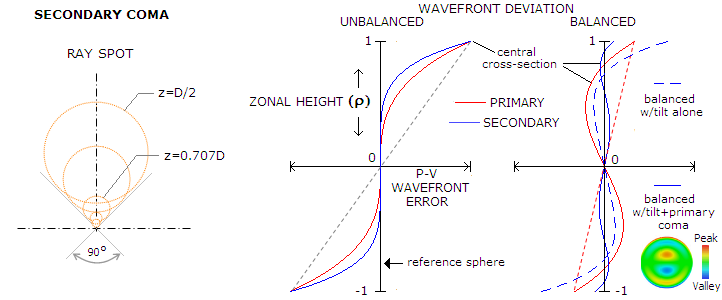FIGURE 42: Ray spot for secondary coma is wider than that for primary coma, with the length-to-width ratio 1.25 vs. 1.5 (left; z stands for zonal height). However, since its deviation increases at a faster rate (5th vs. 3rd power of zonal height), its unbalanced form has smaller overall deviation for given P-V wavefront error, with the RMS wavefront error smaller by a factor of 0.78 (middle). Also, since it can be balanced not only with tilt, like primary coma, but also with primary coma as well, its balanced form has the P-V and RMS wavefront error reduced by a factor of 0.1 and 0.21, respectively.

According to Wcs=Csρ5cosθ (subscript s for "secondary"), for given value of the peak aberration coefficient, the P-V wavefront of secondary coma at the Gaussian focus is identical to the P-V wavefront error of primary coma, but the RMS wavefront error is smaller by a factor of 0.78. When balanced with wavefront tilt, the P-V error Wcst=Cs5-0.5ρ)cosθ is reduced by a factor of 0.5. But it is further reduced by balancing it with primary coma, to Wcstp=Cs5-1.2ρ3+0.3ρ)cosθ, for ρ=1 (both reversal points, at ρ=0.31 and ρ=0.79 radius, have smaller deviation), or 1/10 of its magnitude at the Gaussian focus.

In ray tracing, balancing secondary coma, if significant, is a part of optimization. After cancelling lower-order coma in the 3rd order (for transverse ray, 4th order on the wavefront) approximation, residual higher-order (5th transverse, 6th on the wavefront, or secondary) coma is minimized by reintroducing lower-order coma of opposite sign, with the coefficient (absolute value) larger by a factor of 1.2. As mentioned, the combined RMS wavefront error is smaller by a factor of 0.21 than for unbalanced secondary coma. This means that, similarly to the secondary spherical aberration, secondary coma cannot be entirely removed by balancing it with the lower-order form, only minimized. However, since the magnitude of unbalanced secondary coma is typically relatively low, and both, primary and secondary coma have the same rate of increase (with the square of field angle), the level of balanced coma is usually negligible.

▐    4.3. Astigmatism